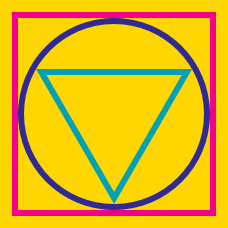Geometry

# Incircle of Triangle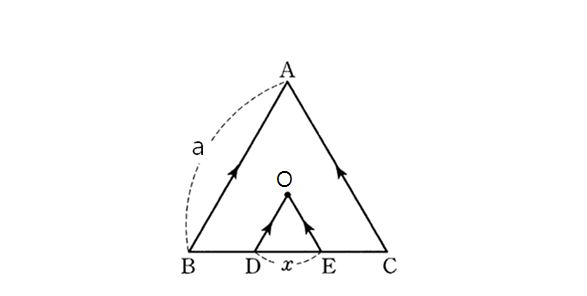In the above diagram, $\triangle ABC$ is an equilateral triangle and point $O$ is the center of the circle inscribed in $\triangle ABC.$ If the length of $\overline{AB}$ is $\lvert \overline{AB} \rvert = 27,$ $\overline{AB} \text{ and } \overline{OD}$ are parallel, and $\overline{AC} \text{ and } \overline{OE}$ are parallel, what is $\lvert\overline{DE}\rvert(=x)?$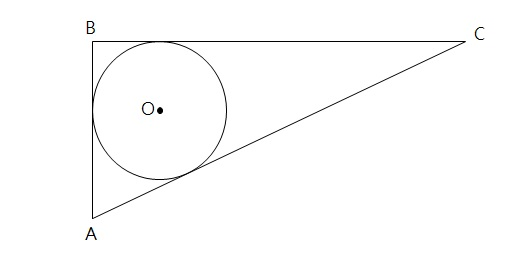In the above diagram, circle $O$ is the incircle of right triangle $ABC.$ Given the two side lengths $\lvert\overline{AB}\rvert = 7 \text{ and }\lvert\overline{BC}\rvert = 16,$ what is the area of the circle?

Note: The above diagram is not drawn to scale.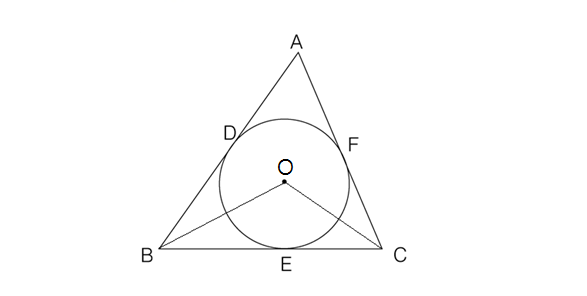In the above diagram, circle $O$ is inscribed in triangle $\triangle ABC,$ with points of contact $D, E,$ and $F$. If $\angle DBE = 54^{\circ} \text{ and } \angle DAF = 46^{\circ},$ what is $\angle BOC?$

Note: The above diagram is not drawn to scale.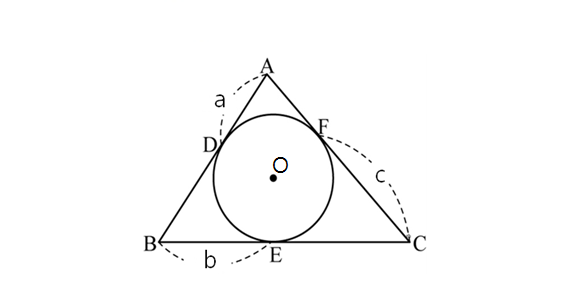In the above diagram, circle $O$ is inscribed in $\triangle ABC$, with points of contact at points $D, E$ and $F$. If $a=4, b=7$ and $c=9,$ what is the perimeter of triangle $\triangle ABC?$

Note: The above diagram is not drawn to scale.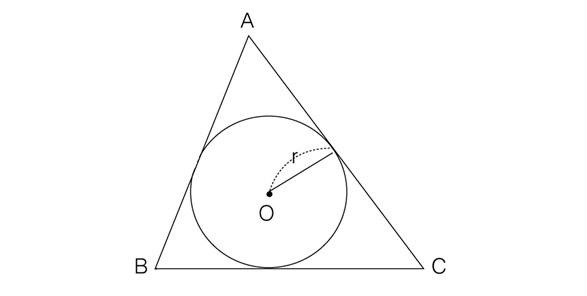In the above diagram, circle $O$ with radius $r=20$ is inscribed in $\triangle ABC$. If the perimeter of $\triangle ABC$ is $x$ and the area of $\triangle ABC$ is $y$, what is $\frac{y}{x}?$

×